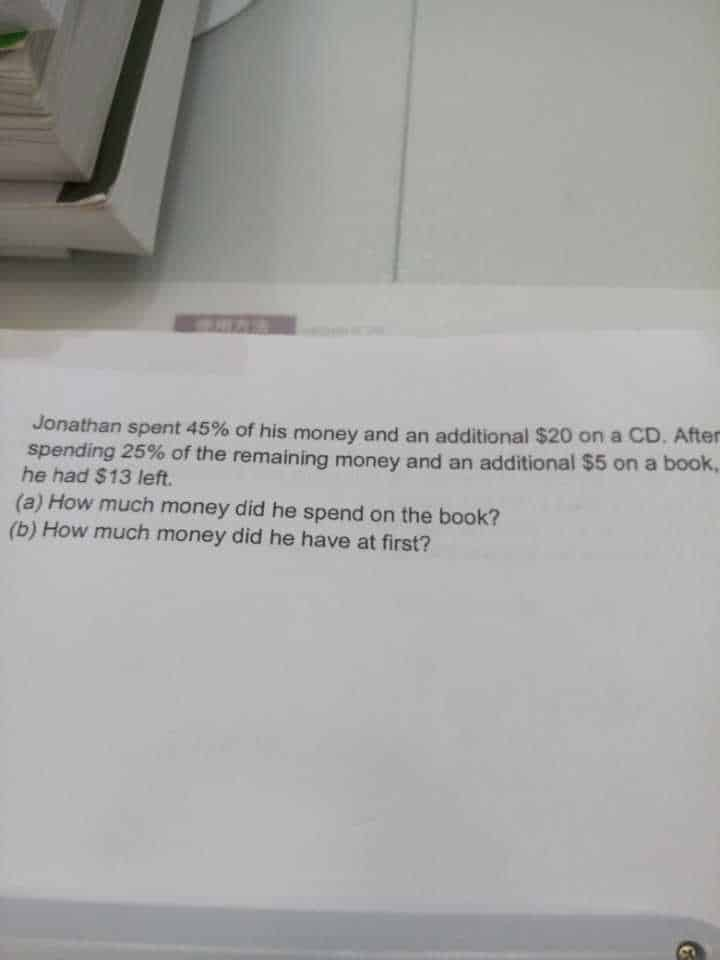# QuestionAnother help. Thanks

(a) \$13+\$5= \$18

This \$18 makes up 75% of the remaining money.

\$(18/75)*25 =\$6  (25% of remaining money)

Amount he spent on book = \$6 + \$5 = \$11

(b) Amount after he buy CD = \$11 + \$13 = \$24

55% of his money = \$24 + the additional \$20 he used to buy CD = \$44

100% = \$(44/55)*100 = \$80

Amount of money he have at first = \$80

0 Replies 0 Likes